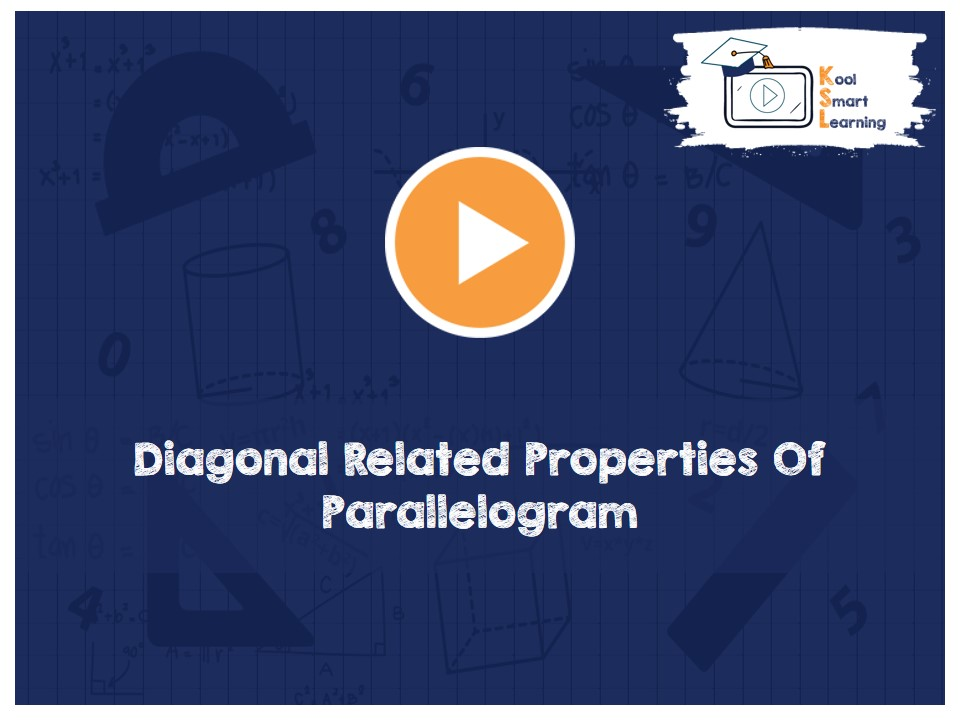At KoolSmartLearning, we intend to harness the power of online education to make learning easy.## Diagonal Related Properties Of Parallelogram

/  Diagonal Related Properties Of Parallelogram## Diagonal Related Properties Of Parallelogram

This video talks about Diagonal related Theorems of the parallelogram. The proof of each one of the theorems is also explained in the video. The theorems covered in this video are – A diagonal of parallelogram divides it into two congruent triangles, the diagonals of a parallelogram bisect each other and its converse theorem (If the diagonals of a quadrilateral bisect each other then it is a parallelogram).

More Related Videos